# 635 Some Multiplication Facts to Know Backwards and Forwards

Something amazing happens in the multiplication table when an even number is multiplied by 6.

Something just as amazing (but backwards) happens in the multiplication table when a multiple of 3 is multiplied by 7.

Here is a graphic that may be helpful for students memorizing some of those 6 and 7 multiplication facts. It is meant to be read from left to right and contains some cool coincidences: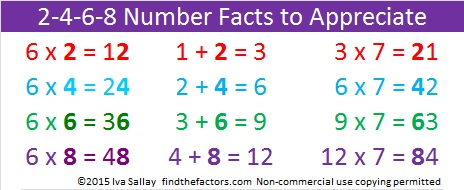Knowing those 6 and 7 multiplication facts will help any student know the multiplication table backwards and forwards!

While it isn’t necessary to memorize the following number facts, some of the patterns in the graphic above continue with them:

• 10 + 5 = 15, and 15 x 7 = 105
• 12 + 6 = 18, and 18 x 7 = 126
• 14 + 7 = 21, and 21 x 7 = 147
• 16 + 8 = 24, and 24 x 7 = 168
• 18 + 9 = 27, and 27 x 7 = 189

———————————————————

What makes 635 an interesting number?

635 is the sum of the nine prime numbers from 53 to 89.

It is also the sum of the thirteen prime numbers from 23 to 73.

635 can be written as the sum of 5 consecutive numbers:

125 + 126 + 127 + 128 + 129 = 635.

635 is a part of exactly 4 Pythagorean triples. Which factors of 635 are greatest common factors for the non-primitive triples?

• 635 is the hypotenuse of the Pythagorean triple 381-508-635
• 635 is the short leg in the triple 635-1524-1650
• 635 is the short leg in the triple 635-40320-40325
• 635 is the short leg in the primitive triple 635-201612-201613

———————————————————————–

• 635 is a composite number.
• Prime factorization: 635 = 5 x 127
• The exponents in the prime factorization are 1 and 1. Adding one to each and multiplying we get (1 + 1)(1 + 1) = 2 x 2 = 4. Therefore 635 has exactly 4 factors.
• Factors of 635: 1, 5, 127, 635
• Factor pairs: 635 = 1 x 635 or 5 x 127
• 635 has no square factors that allow its square root to be simplified. √635 ≈ 25.199206.# 199 My List of Multiplication Rhymes

• 199 is a prime number.
• Prime factorization: 199 is prime.
• The exponent of prime number 199 is 1. Adding 1 to that exponent we get (1 + 1) = 2. Therefore 199 has exactly 2 factors.
• Factors of 199: 1, 199
• Factor pairs: 199 = 1 x 199
• 199 has no square factors that allow its square root to be simplified. √199 ≈ 14.1067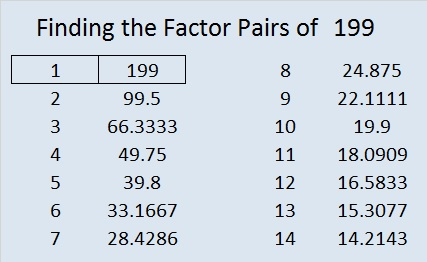How do we know that 199 is a prime number? If 199 were not a prime number, then it would be divisible by at least one prime number less than or equal to √199 ≈ 14.1. Since 199 cannot be divided evenly by 2, 3, 5, 7, 11, or 13, we know that 199 is a prime number.

———————————

I recently wrote a post that asked “Are Multiplication Rhymes Able to Help Kids Learn the Multiplication Table?” Here is a list of rhymes that I’ve put together.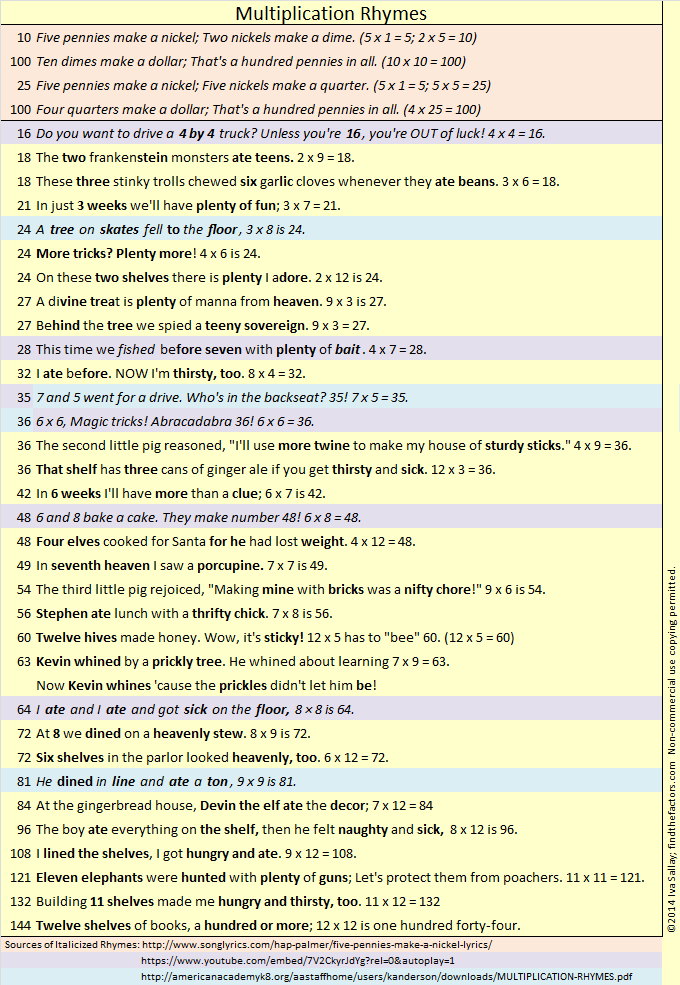Most of the rhymes have rhyming clues to help students remember both numbers being multiplied as well as their product. I wrote the rhymes that are highlighted in yellow. Those highlighted in peach, purple, or blue are from other sources, but I did alter the rhymes for 24, 28, and 81. A black and white pdf of the rhymes is also available here.

# 17 Christmas Angels

• 17 is a prime number.
• Prime factorization: 17 is prime.
• The exponent of prime number 17 is 1. Adding 1 to that exponent we get (1 + 1) = 2. Therefore 17 has exactly 2 factors.
• Factors of 17: 1, 17
• Factor pairs: 17 = 1 x 17
• 17 has no square factors that allow its square root to be simplified. √17 ≈ 4.123.How do we know that 17 is a prime number? If 17 were not a prime number, then it would be divisible by at least one prime number less than or equal to √17 ≈ 4.1. Since 17 cannot be divided evenly by 2 or 3, we know that 17 is a prime number.

17 is never a clue in the FIND THE FACTORS puzzles.

Many Christmas trees in the United States have been up and decorated for weeks. Some of them have a beautiful angel on the top to remind us of the angel that visited the shepherds. In Hungary, the angel is remembered in a different way. There the Christmas tree is put up on Christmas Eve. Tradition says that angels are the ones who decorate the tree with the delicious candies called szaloncukor. The candies are wrapped in specially prepared white tissue and fastened to the tree with white yarn. See the related articles at the end of the post for more information about this fascinating tradition.

The angel puzzles that I’ve made for this post have a few extra clues so they will be easier to solve. The first level 5 puzzle even has many of the same clues as the level 4 puzzle. Nevertheless, be careful because each level 5 angel has a few tricks up her sleeve. Still if you can write the numbers 1 to 12 in both the top row and the first column so that those numbers are the factors of the given clues, then you’ve solved the puzzle. There is only one solution to each puzzle. Click 12 Factors 2013-12-19 for a printable version of these and a few other puzzles.

Hungary:

United States:

# 4 Rhyme and Rhythm

4  is the first composite number, and it is 2 squared. 4 = 1 x 4 or 2 x 2. Factors of 4: 1, 2, 4. Prime factorization: 4 = 2 x 2, which can also be written 4 = 2².

Since √4 = 2, a whole number, 4 is a perfect square.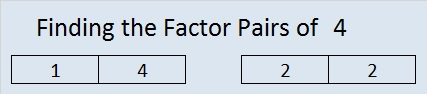When 4 is a clue in the FIND THE FACTORS puzzles, the factors might be 1 and 4 or they might be 2 and 2.

—————————————————————–

Seventeen years ago, when my daughter was learning the multiplication facts, I came across a rhyme that taught one fact:

5, 6, 7, 8……….56 is 7 times 8.

Coincidentally,

1, 2, 3, 4……….I know twelve is 3 times 4.

3 x 4 = 12 isn’t as difficult to remember as 7 x 8 = 56. Still I find it fun to notice the relationship between counting to eight and these two multiplication facts. I enjoyed the rhymes mentioned in my 10/31/2013 post, and I have found yet another site with rhymes for learning the multiplication facts. Two rhymes similar to these 12 and 56 counting rhymes were even included!  The site is : http://www.teacherweb.com/NY/Quogue/MrsLevy/MULTIPLICATION-RHYMES.pdf

Some children have no problem memorizing number facts, but for some children, a rhyme makes learning the facts more fun and much easier.  Even though I already know all of the basic multiplication facts, I am going to memorize these rhymes simply because I personally enjoy them.  I also know I will have at least one opportunity every week to share them with someone trying to memorize the facts: Already when a student asks me, “what’s 7 x 8?” I always answer in rhyme.

Besides the two rhymes listed above, my favorites are:

 Times One: Mirror, mirror look and see, it’s the other number, not me. Times Zero:  Zero is always the hero Six times six / Magic tricks / Abracadabra / thirty-six A tree on skates fell on the floor / Three times eight is twenty-four. A 4 by 4 is a big machine, Iʼm going to get one when Iʼm 16.

This week I even wrote one myself:  Twelve times twelve / Is a dozen dozen / A gross one forty-four / Just ask my cousin.

When a very young child loves a rhyme, he or she will want to hear it over and over again. Mother Goose rhymes have been enriching lives for years. Being able to fully comprehend what the rhyme is about isn’t necessary at first so even preschoolers can be introduced to these rhymes.

Robin Liner writes a blog (crazygoodreaders.wordpress.com) that discusses reading and dyslexia.  On October 5, 2013 , she wrote Rhythm and Rhyme: A Phonological Power Tool.  She wrote, “Rhymes provide subconscious clues.” That means someone is more likely to get an answer right when that answer rhymes with the question. What a fun and powerful way to learn!

Much of what she wrote not only applies to learning to read but also to learning math, science, history, ……. anything.

Twice a week I put 6 new puzzles in an excel file that is attached to this blog. The puzzles can be solved using logic and knowledge of the multiplication table. Here is one of the puzzles I created this week.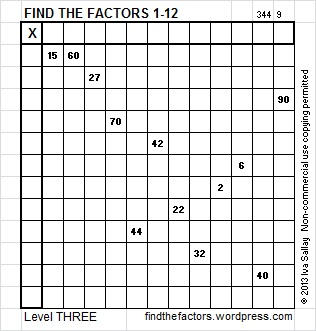Click on this link, 12 Factors 2013-11-07,  for more puzzles and the previous week’s solutions. How do you solve the puzzles? Place the numbers 1 – 12 in both the top row and the first column so that those numbers are the factors of the given clues.

Update: I like the idea of using rhymes to learn multiplication facts so much that I compiled my own list:# 2 Gross Multiplication FactsThis level TWO multiplication table is only a little more difficult than the table in my previous post.  This puzzle uses 12 factors while the previous one used just 10. To find all these factors you have to be familiar with 144 multiplication facts, a requirement that sounds much more intimidating than it really is. (For example, 3 x 5 and 5 x 3 are counted as 2 different facts.) 144 is a fascinating number.  It is a dozen dozen, otherwise known as a gross. Therefore, completing this puzzle will help you review the gross multiplication facts!   If you haven’t memorized all of the facts, there is a gross way to learn some of the gross multiplication facts.

Edwin A. Anderson Elementary School uses manipulatives and rhymes to teach the multiplication facts.  Their students learn two rhymes a week for sixteen week and are masters of the gross multiplication facts when they are done. Five of the rhymes are actually a little gross:

• 3×3, nine cuts on my knee
• 3×8, 24 horseflies on my plate
• 4×7, 28 spiders are webbin’
• 6×8, fishing bait, count slimy worms all 48
• 7×7, roaches on a vine, yucky and scary all 49

It was a very gross multiplication rhyme about the number 64 that inspired me to collect some favorite rhymes and even write a few rhymes myself. I collected them in one list I titled Multiplication Rhymes:That gross, but inspiring, rhyme about the number 64 and some other gross multiplication rhymes are listed below:

• 18 The two Frankenstein monsters ate teens. 2×9=18
• 18 These three stinky trolls chewed six garlic cloves whenever they ate beans. 3×6=18
• 36 That shelf has three cans of ginger ale if you get thirsty and sick. 12×3=36
• 63 Kevin whined by a prickly tree. He whined about learning 7×9=63. Now Kevin whines ’cause the prickles didn’t let him be!
• 64 I ate and I ate and got sick on the floor, 8×8 is 64.
• 96 The boy ate everything on the shelf. Then he felt naughty and sick. 8×12 is 96.

Knowing all 144 (gross) multiplication facts will help you complete my puzzles.

As you Find the Factors and fill in those gross multiplication facts, there are two things I’d like you to remember:

1. Don’t write anything on the inside of the puzzle until you write down all the factors on the outside of the puzzle.

2. The only numbers allowed in the factor row (top row) and the factor column (1st column) are the numbers from 1 to 12, and all 12 numbers must appear in both places.

Now you can click on 12 Factors 2013-10-31, to find the 6 puzzles appearing in this post.

Now let’s think about the number 2:

2 is the smallest prime number and the only prime that is an even number.

2 is also the largest number that is equal to its number of factors.

In particular situations, there are several prefixes and words that mean two:

• 2 is the second counting number.
• A bicycle is a two-wheeler. A binomial is a polynomial with two terms.
• Two musicians form a duet. Take a look at duet’s synonyms and translations in other languages.
• The playing card with a 2 on it is called the deuce.
• A pair can be two shoes, two eyes, or lots of other possibilities.
• Two people in a relationship are called a couple.

Can you think of any more?

Base 2 uses only the digits 1 and 0 to represent each counting number. Computers use base 2, or binary, as it is also called.

Wikipedia informs us of a Calculus topic about the number 2:

• If you take the sum from 0 to ∞ of b⁻ᵏ, it will converge to b, ONLY when the base number, b, is 2. Thus,
•  ⅟₁ + ⅟₂ + ⅟₄ + ⅟₈ + ⅟₁₆ + ⅟₃₂ + ⅟₆₄ + ⅟₁₂₈ + … = 2

Here is 2’s factoring information:

• 2 is a prime number.
• Prime factorization: 2 is prime.
• The exponent of prime number 2 is 1. Adding 1 to that exponent we get (1 + 1) = 2. Therefore 2 has exactly 2 factors.
• Factors of 2: 1, 2
• Factor pairs: 2 = 1 x 2
• 2 has no square factors that allow its square root to be simplified. √2 ≈ 1.414.How do we know that 2 is a prime number? If 2 were not a prime number, then it would be divisible by at least one prime number less than or equal to √2 ≈ 1.4. Since there are no prime numbers less than or equal to 1.4, we know 2 is a prime number.

When 2 is a clue in the FIND THE FACTOR puzzles, the factors are always 1 and 2.